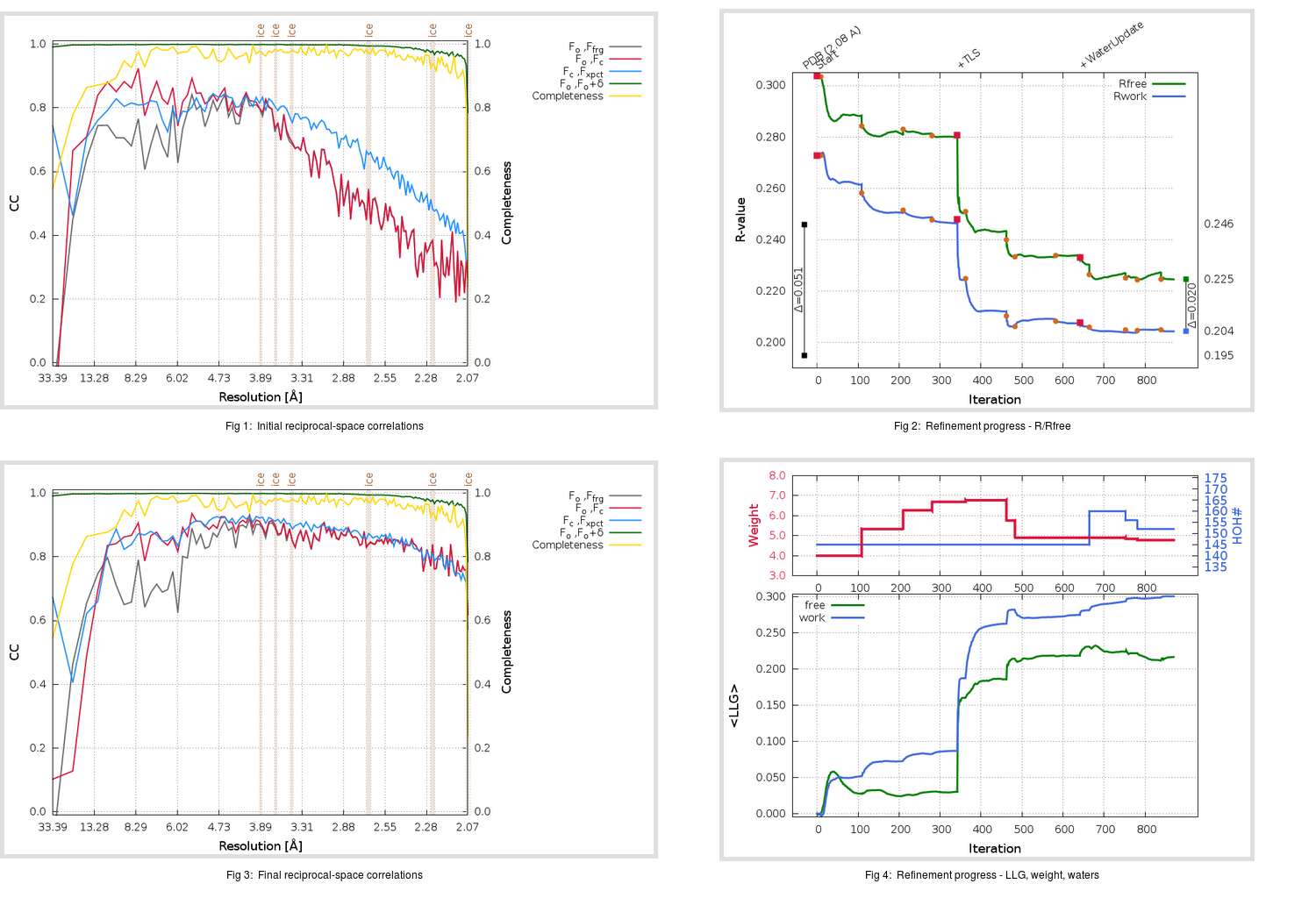Content:

```    Diffraction limits & principal axes of ellipsoid fitted to diffraction cut-off surface:
2.053         0.8129   0.0000   0.5824       0.978 a* + 0.210 c*
2.040         0.0000   1.0000   0.0000       b*
1.995        -0.5824   0.0000   0.8129      -0.784 a* + 0.621 c*
```

## Deposited

` `
 Date deposited Date data collection Resolution R, Rfree 20050630 20041117 2.08 0.1920 0.2460

Molprobity (CCP4 7.0 version) summary:

```Ramachandran outliers =   1.64 %
favored =  92.43 %
Rotamer outliers      =   7.94 %
C-beta deviations     =    16
Clashscore            =  18.74
RMS(bonds)            =   0.0293
RMS(angles)           =   2.81
MolProbity score      =   2.92
Resolution            =   2.08
R-work                =   0.1920
R-free                =   0.2460
```

```Number of waters      =   145

<B> (all atoms) =   56.68 ( sd =    9.51 ) for       2582 non-hydrogen atoms
<B>   (protein) =   56.08 ( sd =    9.08 ) for       2431 non-hydrogen atoms
<B>     (water) =   66.36 ( sd =    9.14 ) for        145 non-hydrogen atoms
<B>    (others) =   58.70 ( sd =   33.84 ) for          6 non-hydrogen atoms

B min/max       (all non-hydrogen atoms) =   30.99 /   97.41
B min/max   (protein non-hydrogen atoms) =   30.99 /   97.41
B min/max     (water non-hydrogen atoms) =   40.54 /   89.97
B min/max     (other non-hydrogen atoms) =   35.18 /   82.03
```

## BUSTER (re-)refinement

` `

Molprobity (CCP4 7.0 version) summary:

```Ramachandran outliers =   0.33 %
favored =  97.04 %
Rotamer outliers      =   5.05 %
C-beta deviations     =     1
Clashscore            =   4.12
RMS(bonds)            =   0.0116
RMS(angles)           =   1.61
MolProbity score      =   1.90
Resolution            =   2.06
R-work                =   0.2044
R-free                =   0.2246
```

```Number of waters      =   152

<B> (all atoms) =   60.94 ( sd =   15.59 ) for       2589 non-hydrogen atoms
<B>   (protein) =   60.32 ( sd =   15.15 ) for       2431 non-hydrogen atoms
<B>     (water) =   70.71 ( sd =   17.71 ) for        152 non-hydrogen atoms
<B>    (others) =   59.34 ( sd =   38.35 ) for          6 non-hydrogen atoms

B min/max       (all non-hydrogen atoms) =   32.07 /  187.34
B min/max   (protein non-hydrogen atoms) =   32.07 /  105.87
B min/max     (water non-hydrogen atoms) =   33.69 /  187.34
B min/max     (other non-hydrogen atoms) =   33.52 /   90.23
```

Refinement progression:Results:

` `
 File Remark 2A5A_aB_refine.01_03_refine.pdb.gz exact refinement commands are in header 2A5A_aB_refine.01_03_refine.mtz.gz including original deposited data and several re-refinement map coefficients 2A5A_aB_refine.01_03_BUSTER_model.cif.gz including any non-standard compound restraints 2A5A_aB_refine.01_03_BUSTER_refln.cif.gz